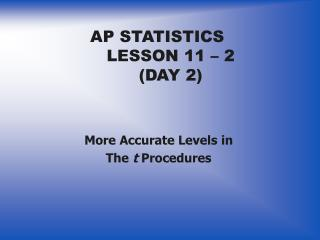DownloadDownload PresentationAP STATISTICS LESSON 11 – 2 (DAY 2)

# AP STATISTICS LESSON 11 – 2 (DAY 2)

Download Presentation## AP STATISTICS LESSON 11 – 2 (DAY 2)

- - - - - - - - - - - - - - - - - - - - - - - - - - - E N D - - - - - - - - - - - - - - - - - - - - - - - - - - -
##### Presentation Transcript

1. AP STATISTICSLESSON 11 – 2(DAY 2) More Accurate Levels in The t Procedures

2. ESSENTIAL QUESTION: How are degrees of freedom figured more accurately and what procedures are used to find two-sample confidence intervals and significance tests in the calculator? Objectives: • To contrast the two methods of calculating degrees of freedom for two-sample t tests. • To use the calculator to find two-sample T tests and intervals.

3. More Accurate Levels in the t Procedure • The two-sample t statistic does not have a t distribution. • Moreover, the exact distribution changes as the unknown population standard deviations σ1 and σ2 change.

4. Approximate Distribution of the Two-sample t statisticPage 659 t procedures remain the same. The degree of freedom is the only thing that changes.

5. Example 11.13 Page 659Calcium and Blood Pressure, continued Two-sample Inference Use Technology Toolbox on page 660.

6. Example 11.14 Page 662DDT Poisoning The calculations using the more complex methods of finding the degrees of freedom are best used by the calculator. The difference is rarely of practical importance.

7. The Pooled Two-sample Procedures • Procedures that average use the statistical term “pooled.” • Pooled two-sample t procedures is a situation where the variances of both the samples are assumed to be the same and the sample sizes are the same. This rarely happens and the same results will occur with regular t procedures. • On the print out from a computer use the unequal line for variances, degrees of freedom, and probability.